Journal of Finance and Accounting
Volume 3, Issue 3, May 2015, Pages: 35-41

Post-Earnings Announcement Drift: The Role of Earnings Volatility

Ben Mhamed Yosra, Jilani Fawzi

Department of finance, Faculty of Economic sciences and Management of Tunis, University of Tunis El Manar, Tunisia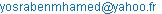(B. M. Yosra)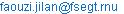. tn (J. Faouzi)

Ben Mhamed Yosra, Jilani Fawzi. Post-Earnings Announcement Drift: The Role of Earnings Volatility. Journal of Finance and Accounting. Vol. 3, No. 3, 2015, pp. 35-41. doi: 10.11648/j.jfa.20150303.11

Abstract: The study reported here consisted of examining the market’s reactions to the volatility effect on time series correlations of earnings in a post-earnings announcement drift context. Sample in this study comprises of 295 Canadian firms and covers 2006-2011 period. Firstly, our results show that earnings volatility is inversely related to earnings persistence (under the AR(1) and the Foster model assumption). Secondly, our findings confirm the aggravated negative effect of earnings volatility on seasonal unexpected earnings persistence. Finally, following Mishkin’s (1983) method of testing market efficiency, this study supports that capital market recognizes the earnings volatility effect on earnings persistence. Our results contribute to understanding the role of earnings volatility in explaining the persistence of PEAD.

Keywords: Earnings-Announcement Drift, Earnings Volatility, Standardized Unexpected Earnings

1. Introduction

Several studies have documented that past earnings volatility reduces earnings predictability. Graham et al. (2005) present managers’ viewpoints of the negative relation between earnings persistence and volatility and their preference for smoother reported earnings. Other subsequent works provide empirical proof for these widely held belief (Dichev et Tang, 2009 ; Frankel et Litov, 2009; Petrovic et al, 2009 ; Hamzavi et Aflatooni, 2011; khodadadi et al, 2012 ; Cao et Narayanamoorthy, 2012). They show that the variability contains incremental information that improves the prediction of future firm performance. More important, Minton et al. (2002) argue that investors do not understand information on volatility in equity valuation. Likewise, Dichev and Tang (2009) demonstrate that analysts’ forecasts do not fully incorporate the information contained in earnings volatility. Specially, they show that analysts appear to ignore predictable implications of earnings variability for future earnings. Contrary of their tests, Frankel and Litov (2009) conduct a stock market based tests. They argued that investors do not underestimate the effects of earnings volatility, but they do not verify the market expectation. In this review of volatility implications literature, Cao and Narayanamoorthy (2012) demonstrate that the market systematically underestimates the time-series properties resulting from earnings volatility; otherwise known as Post-Earnings announcement drift anomaly1. This paper investigates the possibility that stock prices reflect fully the implications of volatility for time-series behavior of earnings.

Several studies argue the existence of a PEAD, in which the market ignores the serial correlation in standardized unexpected earnings (SUE). Beginning with Ball and Brown (1968), the financial literature exploit a setting in which stock return continue to move in the same direction as the earnings surprise. According to Bernard and Thomas (1990), the PEAD anomaly has centered around the failure of stock prices to reflect the implication of current earnings for future earnings. Specifically, they find that investors do not fully exploit the past time series properties of the quarters series. Narayanamoorthy (2006) extends the finding of Bernard and Thomas by exploiting a new cross sectional setting. He shows that the market ignore SUE autocorrelation resulting from accounting conservatism. Recently, Cao and Narayanamoorthy (2012) argue that investors tend to underreact to the effects of earnings volatility for SUE autocorrelation; generating PEAD abnormal return.

In this study, we investigate if investors underreact to the information content of earnings volatility. Otherwise, our analyses explore the implications of earnings volatility for PEAD abnormal return. First, we hypothesize that earnings volatility is inversely related to quarterly earnings persistence. We find that earnings volatility has a negative effect on earnings persistence and earnings predictability, which corroborate the result of Dichev and Tang (2009). The persistence coefficient declines from 0.785 in the lowest quintile of volatility to 0.311 in the highest quintile of volatility. Second, we investigate whether investors understand the implications of earnings volatility on earnings surprises persistence. Our approach is to verify if the variation in the abnormal return mirrors the variation in SUE autocorrelation. We find that the abnormal return pattern is not similar to the autoregressive coefficient pattern. Specifically, we observe that the abnormal return increases by 0.41% as we move from the lowest decile of volatility to the highest decile. This variation is different in magnitude to that in SUE autocorrelation. We also conduct formal tests of whether the signs and magnitude of abnormal returns reflect the implications of volatility for the Sue autocorrelation structure. Our findings reject the hypothesis that the market uses a naive seasonal random walk model to form quarterly earnings expectations.

2. Prior Research and Motivation

In this section, first we explore the link between earnings volatility and persistence. Then, we focus on PEAD type of study which identify the market’s reaction to the earnings surprises. Finally we develop hypothesis.

2.1. Earnings Volatility Effect

The volatility of reported earnings is the result of economic shocks and of problems in accounting determination of income. So that, we view that earnings volatility is arising essentially from uncertainty of operations and accounting choices. Both of these factors become part of the permanent earnings series, and reduce the earnings persistence and the earnings predictability.

On the literature review, to my knowledge Minton et al. (2002) is the first research that tests the impact of volatility on future earnings « …, none have considered the role of volatility in forecasting levels of future cash flows or earnings» (Minton et al., 2002, pp 196). The authors find evidence that current cash flows (earnings) are inversely related to future cash flows (earnings). They suggest that this finding is consistent with the underinvestment explication. The effect of volatility on underinvestment is the fact that high volatility increases (1) the cost of external capital, (2) the internal cash flows shortfalls. Moreover, they empirically document that forecasting model that incorporate earnings volatility is better than forecasts from models that exclude volatility, in term of lower forecasts error and less biased predictions.

Lastly and more importantly, prior literature also examines the relation between earnings volatility and earnings predictability, which is more relevant to our study. There is a lack of evidence regarding how accounting volatility affects earnings predictability. « Our knowledge about predictability is limited » (Dichev and Tang, 2009, p161). The recent survey evidence from research of Graham et al. (2005) motivates Dichev and Tang to test the validity of these beliefs. Graham et al. survey 401 managers and find that 97% of respondents prefer smooth earnings. 80% of these managers pronounced aversion to earnings volatility because they believe that it reduce the predictability of earnings. To enhance the knowledge in this area, Dichev and Tang decide to analyze this link and to provide empirical evidence about it.

They argue that earnings volatility is inversely related to earnings persistence and to earnings predictability. They formed quintiles on earnings volatility, and documents across quintile portfolios that the persistence coefficient declines from 0.93 in the low quintile to 0.51 in the high quintile. Likewise, low volatility earnings have much high coefficient of predictability as compared to high earnings volatility (0.3 vs 0.7). They argue that two factors combine to predict this negative relationship: economic shocks and problems in the accounting determination of reported income. Further, they study whether the financial analysts are aware of the existence of the relation between ex-ante volatility and future earnings persistence. The work of Dichev and Tang has been a staple in this area. Several empirical researches have related this study to the works that we are going to see.

Frankel and Litov (2009) believe that Dichev and Tang address an interesting and relevant issue in which there is little evidence. So, they revisit their findings to provide evidence that supports the existence of a relationship -earnings volatility and earnings persistence- and to verify whether investors completely understand the effects of earnings volatility. They conclude that with additional controls tests the relationship is still robust, and that investors do not underestimate it.

In a similar vein, Hamzavi and Aflatooni (2011) analyses the effect of the income smoothing behavior (inverse proxy of earnings volatility) on earnings persistence and earnings predictability. Similar to previous research and using the same empirical test (quintile test), they find that the earnings predictability and earnings persistence of smoothers is higher than that of other firms. Moreover, more recent literature suggests strong evidence of the negative effect of earnings volatility on earnings predictability. Interestingly, Cao and Narayanamoorthy (2012) extend the analyses to quarterly earnings. Recently, in 2012, Khodadadi and al. lead a study that fits in the line of research driven by Dichev and Tang (2009). They pushed further their research by focusing on the forecasting ability of accounting income volatility and its components (cash flows volatility and accruals volatility). The empirical results imply that the volatility in earnings is more important in the relation to earnings persistence, than cash flows volatility and accruals volatility. The negative relationship has a remarkable differentiating power in the long horizon of prediction (5 years).

Petrovic and al. (2009) examine the relation between ex-ante volatility and future firm performance. They find that ex-ante earnings volatility is inversely related to future expected earnings. More importantly, they show that this link is more pronounced for the highest earnings firms.

2.2. Market Efficiency Studies: PEAD Context

In the last 40 years, an extensive amount of literature analyses anomalies in the capital market. One of the most puzzling market anomalies, that are dependent on earnings surprises, is the post earnings announcement drift (PEAD) (Bird et al, 2013). Previous research (Foster et al., 1984; Bernard and Thomas, 1990; Ball and Bartov, 1996; Rangan and Sloan, 1998; Soffer and Lys, 1999) show that PEAD is due to naive investors’ failure to recognize the time-series properties of earnings; stock returns continue to drift in the direction of quarterly earnings surprises for the time following an earnings announcement. In other words, if a firm announces, in quarter t, positive (negative) surprise the market tend to be positively (negatively) surprised in quarter t+1. Several studies document that standardized unexpected earnings2 (or earnings surprises) in quarter t is positively correlated to the SUE for adjacent quarters (t-1 to t-3); but this correlation become negative in quarter t-4 (Foster, 1977; Bernard and Thomas, 1990; Bartov, 1992; Ball and Bartov, 1996). The PEAD literature finds that the market does not revise immediately its expectations for future SUE based on quarter’s SUE.

A large amount of studies document that irrational behavior of investors are the main cause of the PEAD existence. Bernard and Thomas (1990) suggest that PEAD happens because investors underreact to earnings news, when expected earnings follow a seasonal random walk. The random walk occurs when return do not dependent on previous returns. Their result imply that after a positive (negative) earnings surprises, subsequent earnings surprises tend to be predictably positive (negative).

Ball and Bartov (1996) document that PEAD is the consequence of investors’ mis-estimating the SUE autocorrelation by 50%. Similarly, Soffer and Lys (1999) provide evidence that investors ignore partially the time-series of quarterly earnings. They show that 50% of this information is anticipated prior the first subsequent earnings announcement. Other researches provide more powerful test of the SUEs autocorrelation pattern by exploiting the cross-sectional variation. For example, Rangan and Sloan (1998) document that PEAD arise from the integral method of reporting "cross quarter effect". They find that autoregressive coefficient is larger when the quarters used belong to the same fiscal year than for quarters in different fiscal year. Then, they show that investors do not recognize the larger autoregressive coefficients between quarters in the same fiscal year. So, they support the finding of Bernard and Thomas, that states: "the PEAD reflects the investors tendency of anchor a naive seasonal random walk earnings expectation." (Rangan et Sloan, 1998, p.369).

Similar to Rangan and Sloan (1998), the study of Narayanamoorthy (2006) utilizes predictable cross-sectional variation in the autocorrelation SUE to examine variation in PEAD. He demonstrates that investors fail to fully incorporate the differential persistence resulting from accounting conservatism. On other words, the findings indicate that stock prices fail to differentiate the time-series properties arising from conservatism accounting. Likewise, Cao and Narayanamoorthy (2012) exploit more this new cross-sectional setting. They examine the earnings volatility-stock return relation by exploring cross-sectional differences in earnings persistence. Cao and Narayanamoorthy discover that autocorrelation of the SUEs are significantly lower for the top deciles of volatility than for the bottom deciles, consistent with volatile earnings having a greater tendency to mean revert faster than persistent earnings. Consequently, they document a negative correlation between earnings volatility and PEAD.

2.3. Hypothesis Development

Dichev and Tang (2009) explore the link between earnings volatility and persistence in terms of annual data. They use the AR(1) process of annual earnings for the empirical test. However, this process does not seem to characterize quarterly earnings which have more complicated time series properties. For this reason, previous research3 introduce other models that Foster model is the most popular (Brown, 1993). This model concludes that the difference between quarterly earnings and the corresponding quarter in the previous year follow an AR(1) process.

Narayanamoorthy (2006) and Cao and Narayanamoorthy (2012) provide justifications for considering a positive relation between earnings persistence and SUE persistence. They confirm that ex-ante volatility have an inverse effect on the persistence of SUE not only for the time series derived using the Foster model, but also for AR(1) process. They find evidence that the quarterly earnings process is well represented by the AR(1) model for annual earnings (Brown and Han, 2000). Referring to the previous study, we must confirm that the effect of earnings volatility on standardized unexpected earnings continuously exists in our samples. This conjecture leads to the following hypothesis:

H1: earnings volatility has an inverse effect on the persistence of standardized unexpected earnings (SUE).

After testing this hypothesis, we then move to test the price stock valuation process. Market efficiency hypothesis have provided confecting evidence. Dichev and Tang (2009) conclude that analysts cannot understand the implications of earnings volatility for earnings predictability. But, Frankel and Litov (2009) contend that the market recognize correctly the earnings volatility implications in a stock return test. Tan and Sidhu (2012) document that analysts’ forecasts of earnings incorporate information contained in reported earnings volatility only for firms with a high degree of income smoothing. Under a PEAD context, Cao and Narayanamoorthy (2012) find evidence that investors fail to update its expectations to reflect the information in SUE autocorrelations attributable to volatility. In this study, we analyze market’ expectations under a PEAD context. Therefore, we present our hypothesis as follow:

H2: the capital market cannot understand the earnings volatility effect on earnings persistence.

3. Main Empirical Tests

3.1. Sample Selection and Variable Measurement

Quarterly data is obtained from Reuters base. Our sample consists of non-financial firms listed on Toronto stock exchange from 2006 to 2011. Our sample comprises 13,464 firms quarterly observations. The variable used as a measure of standardized unexpected earnings (SUE) is the change in current earnings from the earnings of the corresponding quarter in the previous year. This approach assumes that quarterly earnings follow a seasonal random walk process. We use the previous fiscal quarter’s closing market value as the scaling factor for SUE. We then measure DSUE as the transformed decile ranking of scaled SUE (numbered from 0 through 9). We then divide the decile ranks by 9 and subtract 0.5 we obtain a scaled ranks which vary from -0.5 to +0.5. Because the most drift studies use decile ranks in the regressions, this transformation facilitates comparison of our results to previous research (Bernard and Thomas, 1990; Rangan and Sloan, 1998; Narayanamoorthy, 2006; Livnat and Mendenhall, 2006; Cao and Narayanamoorthy, 2012). Earnings volatility is calculated by taking the standard deviation of the deflator earnings for the most recent twelve quarters (Wei et Zhang, 2006 ; Chen et al, 2008 et Bandyopadhyay, 2011). We also used decile partitions (from -0.5 to +0.5) of earnings volatility for easier comparison with past PEAD findings.

We computed daily abnormal return as the raw daily return minus CRSP value-weighted index return. Referring to Rangan and Sloan (1998), Soffer (1999), Cao and Narayanamoorthy (2012) and Chen (1012), we use abnormal returns primarily from two windows:

(i) A three-day short window, centered on the next earnings announcement date, and

(ii) A one-quarter long window, beginning two days after the current earnings announcement date and ending one day before the next earnings announcement date.

We used size as control variable in the regression because prior studies (Bernard and Thomas, 1990; Bhushan, 1994; Narayanamoorthy, 2006) shown that the drift is correlated with this variable. DSize is the decile rank of the market capitalization at the end of the previous quarter, ranging from -0.5 to +0.5.

Table 1 presents the descriptive statistics of the variables defined previously in our analysis.

As can be seen in this table, the mean SUE is negative, although the median is positive, which is consistent with a higher magnitude of negative earnings surprises. These statistics are similar to those reported in Ball and Bartov (2006) and Jegadeesh and Livnat (2006). In contrast, table 1 reports the mean Vol as positive for our sample, as is the median, which is consistent with sequential volatility increase for most firms. Table 1 also clearly shows that historical data sample has a wide distribution of SUE, VOL and size. By transforming variables into decile ranks, the effect of outliers can be undermined.

Table 1. summary statistics.

 Mean 10% 25% 50% 75% 90% CARS 0.4190 -7.516 -3.383 -0.292 3.2047 8.192 CARl 0.3375 -10.16 -4.111 -0.289 4.0433 11.30 SUE -0.050 -0.411 -0.029 0.0000 0.0280 0.346 VOL 0.0450 0.0057 0.010 0.0223 0.0479 0.092 Size 3188.3 25 89 340 1600 8900

CARS: is the market-adjusted buy and hold return, calculated from the short window. CARL: is the market-adjusted buy and hold return, calculated from the long window. SUE is the difference between the current quarter’s earnings and the earnings of the corresponding quarter in the previous year. VOL is the variance of the most recent twelve quarterly. sizei,t is the market value at the end of the previous quarter.

3.2. Earnings Volatility and Quarterly Earnings Persistence

Following Dichev and Tang (2009) and Cao and Narayanamoorthy (2012), we test the effect of earnings volatility on earnings persistence using Foster’s model1. Since we want to examine the impact of volatility on earnings persistence, we sort the sample into three portfolios according to the level of their earnings volatility in ascending order. So we obtain three quintiles, each containing a third of the population (Q1: the lowest volatility quintile, Q2: the medium volatility quintile, Q3: the highest volatility quintile). For each quintile, we present the persistence coefficient and the R² of regression (the regression of Foster model and AR(1)). These results provide evidence about the economic and statistical significance of the first hypothesis. After replacing seasonal differenced earnings (Foster Model) with a SUE, we obtain the following model: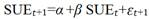(1)

Table 2 presents the persistence coefficient β and the R-squared of this regressions by earnings volatility quintiles.

The persistence coefficient declines from 0.248 in Q1 (the lowest quintile) to 0.187 in Q3 (the highest quintile). The adjusted R-squared declines from 15% to 6%. The table displays also a test of statistical significance of the difference in coefficient of persistence. It’s a simple t-test which indicates that the difference in persistence (6%) between quintile 1 and 3 for earnings volatility are highly significant (p<0.001). The test for difference in R² is a bootstrap test. The test statistic is the difference in adjusted R² between earnings volatility quintile 1 and 3. This test indicates that the difference in R² is highly significant. Therefore, while earnings volatility increases across quintiles, persistence coefficient and adjusted R squared significantly decline.

In cases where Dichev and Tang (2009) conclude that earnings volatility has a negative effect on annual earnings persistence, we aim to extend Dichev and Tang’s volatility effect to quarterly earnings. Thus, in the Panel B of Table 2, we report the persistence coefficient under the AR(1) assumption. The persistence coefficient declines from 0.785 in Q1 to 0.344 in Q3. The adjusted R-squared declines from35.9% to 15.37%. Thus, in this case we conclude that earnings volatility is inversely related to persistence of both quarterly earnings and seasonal differenced earnings.

Table 2. Regression results by quintiles of earnings volatility.

 Panel A: Foster Model Qt+1 - Qt-3=α+ β(Qt-Qt-4) +℮t 𝐷SUE𝑡+1=α +β 𝐷SUE𝑡+℮ 𝑡+1 Earnings volatility β (persistence) Adj R² Quantile 1 (Low) 0.2486** 0.1502 Quantile 2 0.2349*** 0.1606 Quantile 3 (High) 0.1872*** 0.0666 Difference (Q1- Q3) 0.0614*** 0.0836** Panel B: AR(1) Model Qt+1 =α+ βQt +℮t Earnings volatility β (persistence) Adj R² Quantile 1 (Low) 0.7850*** 0.3590 Quantile 2 0.6555 *** 0.3387 Quantile 3 (High) 0.3440 *** 0.1537 Difference (Q1- Q3) 0.441*** 0.2053***

Q is quarterly earnings before extraordinary items. SUE is the difference between the current quarter’s earnings and the earnings of the corresponding quarter in the previous year. VOL is the variance of the most recent twelve quarterly earnings.

*, **, *** : les coefficients sont significatifs aux seuils de 10 %, 5 % et 1 %,

3.3. Market Efficiency Test: Earnings Volatility Effect

To test whether the expectation of investors reflect the information in SUE autocorrelation attributable to volatility, we conduct two sets of tests. Firstly, we investigate the implications of earnings volatility on earnings surprises persistence. Secondly, we verify if the variation in the abnormal return mirrors the variation in SUE autocorrelation. Thus, we follow the model used by Cao and Narayanamoorthy (2012), The regression model is as follows: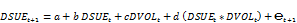(2)

Table 3. Earnings Volatility Effect on Seasonal Difference Earnings Autocorrelation.

 Dependant Variable DSUEt+1 Coefficient Z-stat P > |Z| DSUE 0.370 22.9 0.000 DVOL 0.031 2.32 0.02 DSUE*DVOL -0.085 -1.85 0.065 DSize 0.031 2.26 0.024 DSUE*DSize -0.102 -2.25 0.024

SUE is the difference between the current quarter’s earnings and the earnings of the corresponding quarter in the previous year. 𝐷SUEi,t is the scaled decile rank for each quarter transformed by dividing by 9 and then subtracting 0.5. Thus, 𝐷SUE𝑖, is ranging from -0.5 and +0.5. VOL is the variance of the most recent twelve quarterly earnings. DVOLi,t is the earnings volatility (VOL) decile rank for each quarter transformed by dividing the rank by 9 and subtracting 0.5, resulting in values that range from-0.5 to +0.5. D𝑆izei,t is the decile rank of the market value at the end of the previous quarter, ranging from -0.5 to +0.5 after transformation.

DVOL is the VOL decile ranking for each quarter ranging from -0.5 to + 0.5. DSUE is the earnings surprise measure, defined as in the previous section. To examine the effect of earnings volatility, we used the product of DSUE and DVOL as an independent variable in the regression. The interaction is reasonable when the implicit assumption is that the higher the level of earnings surprise, the greater the effect of volatility’s variable. We include DVOL as a separate independent variable in the regression to eliminate the correlated omitted variable problem. In table 3 we provide the results for the hypothesis that the earnings volatility has an inverse effect on the persistence of standardized unexpected earnings (SUE).

We observe consistently negative coefficients for the earnings surprise-volatility interaction term. This reaffirms that the SUE autocorrelations decrease in ex-ante volatility. for the median earnings volatility firm ( DVOL=0), the coefficient on DSUE has the predicted positive sign (0.37). Then, we observe that this coefficient vary depending on the different level of earnings volatility. For the bottom decile of volatility, the first-order autoregressive coefficient is 0.412 (0.37+0.085/2), but it is only 0.327 (0.37-0.085/2) for those stocks in the top decile. We also conclude that size is negatively related to earnings persistence. This result contradicts Cao and Narayanamoorthy’s (2012) result, as they detect a positive correlation between size and earnings persistence.

In this section, we test whether the capital market can fully reflect the relation between current and future earnings surprise and the effect of earnings volatility on earnings persistence. For this reason, we use an abnormal return tests that mirror the SUE autocorrelation tests. Abnormal return regressions over the short window and the long window, respectively, is estimated as follows: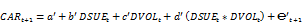(3)

Table 4 presents results of the ability of the capital market to understand the earnings volatility effect on earnings persistence

We expected the middle group of earnings volatility to have positive drift (similar to previous result in table 3). Even so, panel A in table 4 shows that the coefficient on DSUE (DVOL=0) is negative. contrary to what is provided, the median earnings volatility portfolio had a mean drift of -0.18 percent. Firm in the top portfolio had a mean drift of 0.025%(-0.0018+0.0041/2), which is larger than the return of the bottom group of volatility at -0.38%(-0.0018-0.0041/2). A similar picture can be seen in Panel B of table. The average drift is -9 percent for the median earnings volatility group over the long window. The top volatility portfolio had a mean drift of -7% (-0.093+0.0343/2), but the bottom portfolio earn only -11% (-0.093-0.0343/2). The result show a difference with the coefficient of the interaction variable of DSUE regressions (2).

Table 4. earnings Volatility Effect on PEAD Returns.

 Dependant Variable CARt+1 Panel A :3-day returns Panel B : quarterly returns Coefficient Z-stat P > |Z| Coefficient Z-stat P > |Z| DSUE -0.0018 -3.11 0.002 -0.0934 -1.89 0.058 DSUE*DVOL 0.0041 2.41 0.016 0.0343 3.23 0.001 DVOL -0.0022 -3.73 0.000 -0.0097 -2.35 0.019 DSize 0.0018 2.98 0.003 -0.0014 -0.35 0.724 DSUE*DSize 0.0036 2.34 0.020 0.0045 0.41 0.683

CARS: is the market-adjusted buy and hold return, calculated from the short window. CARL: is the market-adjusted buy and hold return, calculated from the long window. SUE is the difference between the current quarter’s earnings and the earnings of the corresponding quarter in the previous year. 𝐷SUEi,t is the scaled decile rank for each quarter transformed by dividing by 9 and then subtracting 0.5. Thus, 𝐷SUE𝑖, is ranging from -0.5 and +0.5. VOL is the variance of the most recent twelve quarterly earnings. DVOLi,t is the earnings volatility (VOL) decile rank for each quarter transformed by dividing the rank by 9 and subtracting 0.5, resulting in values that range from-0.5 to +0.5. D𝑆izei,t is the decile rank of the market value at the end of the previous quarter, ranging from -0.5 to +0.5 after transformation.

Next, we use a market efficiency test that takes the form of the Mishkin test (1983). The objective is to analyze how the market understands the earnings autocorrelation and the effect of earnings volatility in such a process. In this test, a simultaneous equations system are estimated jointly. Firstly, the forecasting equation is identical to equation 2. Secondly, the pricing equation represents the capital market’s response to the forecast error (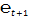) in the forecasting equation. Thus, we estimate the following two equations simultaneously:(4)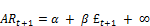(5)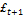in Equation 5 represents the earnings surprise. Under market efficiency, the market expectation of earnings and the earnings volatility effect should equal the expectation that is based on the forecasting equation. The market should react only to the earnings surprise. Otherwise,in Equation 5 should be identical to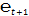in Equation 4. Thus, we substituteinto Equation 4 and get the following: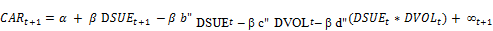(6)

In Equations 4 and 6, 𝑏 and d are the actual coefficients of the current SUE and SUE -volatility interaction term while b’’ and d’’ are the inferred coefficients from the market expectation. Table 5 presents the results simultaneous nonlinear procedure proposed by Mishkin.

We estimate coefficients simultaneously of the two following equations using the simultaneous nonlinear procedure proposed by Mishkin :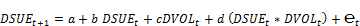(7)(8)

Table 5. Mishkin Test of Market Efficiency for Earnings Volatility Effect.

 3-day returns Quarterly returns Coef P > |Z| Coef P > |Z| b 0.2376 0.0000 0.2733 0.0000 b’’ 0.0021 0.0060 -0.0014 0.2999 d -0.0738 0.0880 -0.1383 0.0186 d’’ -0.1097 0.0605 0.1910 0.8731

Chi-square to Test Market Efficiency Constraints *

 3-day returns Quarterly returns Khi2 P >Chi2 Khi2 P >Chi2 b=b’’ 0.0605 0.8056 0.0047 0.9451 d=d’’ 0.0486 0.8255 0.0912 0.7627

*A significant chi-square value implies that the real coefficient in Equation 4 and the inferred coefficient in Equation 6 are significantly different.

The coefficient of current surprise (b) is positive. The likelihood ratio statistic for the restriction b=b’’ is not significant. The post-estimation test shows that coefficients are different. This result implies that the stock market understands the quarterly earnings process.

In terms of the relation between volatility and SUE autocorrelation, table 5 document two significantly negative Coefficients. Then, the post-estimation test reveals that the market do not underestimates the effect of earnings volatility on SUE persistence with a not significative value of chi-square. Under the PEAD context, we find solid evidence that market recognize SUE autocorrelation and earnings volatility effect on this process. A similar finding is observed by Frankel and Litov (2009), Chen (2012) and Tan and Sidhu (2012).

4. Conclusion

To see if we can accept our hypothesis, if the market understand the effect of earnings volatility on SUE persistence, we have focused to look at the implication of earnings volatility on the correlation between earnings surprise and stock’s abnormal return. In the first phase of this study, we test the relation between earnings volatility and earnings persistence. Our results demonstrate a negative sensibility of earnings persistence to ex-ante volatility. In the second, we show that earnings volatility has an inverse effect on the persistence of standardized unexpected earnings (SUE). Finally, we examine the role of earnings persistence in predicting post announcement abnormal returns. Under the PEAD context, we find solid evidence that market recognize the earnings volatility effect on quarterly earnings process.

Further research could elaborate more on the consequences of earnings volatility and its causes within a bigger picture. There is possibility to dig deeper for the reasons for earnings volatility by testing firms’ characteristics and determine which factor makes market more efficient.

References

1. Ball R. and Bartov E. (1996),« How naïve is the stock market’s use of earnings information? », Journal of Accounting and Economics, Vol.21, n°3, pp.319–337.
2. Ball R. and Brown P. (1968),« An empirical evaluation of accounting income numbers », Journal of Accounting Research, Vol. 6, n°2, pp.159–178.
3. Bandyopadhyay S., Huang A. and Wirjanto T., (2011),« Does Income Smoothing Really Create Value ?», University of Waterloo, Working Paper.
4. Bartov E. (1992),« Patterns in unexpected earnings as an explanation for post-announcement drift », The Accounting Review, Vol .27, n°3, pp.610–622.
5. Bernard V.L. and Thomas J.K. (1990),"Evidence That Stock Prices Do Not Fully Reflect the Implications of Current Earnings for Future Earnings", Journal of Accounting and Economics, Vol.13, n°4, pp. 305-340.
6. Bhushan R. (1994),« An informational efficiency perspective on the post-earnings announcement drift », Journal of Accounting and Economics, Vol.18, pp.45-65.
7. Brown L.D. (1993),"Earnings forecasting research: its implications for capital markets research", International Journal of Forecasting, Vol. 9, pp. 295–320.
8. Cao S.S. and Narayanamoorthy G.S. (2012),« Earnings volatility, Post-Earnings Anouncement Drift and Trading Frictions», Journal of Accounting Research, Vol.50, n°1, pp.41-74.
9. Changling Chen. (2013), "Time-Varying Earnings Persistence and the Delayed Stock Return Reaction to Earnings Announcements", Contemporary Accounting Research, Vol.30, n°2, pp.549–578.
10. Chen C., Huang A.G. and Jha R. (2008),« Trends in Earnings Volatility, Earnings Quality and Idiosyncratic Return Volatility: Managerial Opportunism or Economic Activity », School of Accounting and Finance, University of Waterloo.
11. Dichev I.D. and Tang V.W., (2009),"Earnings volatility and earnings predictability", Journal of Accounting and Economics, Vol.47, pp.160-181.
12. Frankel. and Litov. (2009),« Earnings Persistence », Journal of Accounting and Economics, Vol.47, pp.182-190.
13. Foster G. (1977),"Quarterly accounting data: time-series properties and predictive-ability
14. results", The Accounting Review, Vol.52, pp.1–21.
15. Foster G., Olsen C. and Shevlin T. (1984),« Earnings releases, anomalies, and the behavior of security returns », The Accounting Review, Vol.59, n°4, pp.574–604.
16. Graham J., Campbell H. and Rajgopal S. (2005),"The economic implications of corporate financial reporting", Journal of Accounting and Economics, Vol.40, pp.3–73.
17. Hamzavi M.A. and Aflatooni A. (2011),« Earnings Smoothing and Earnings Predictability », Business Intelligence Journal, pp. 187-201.
18. Khodadadi V., Tamjidi N., Fazeli Y.S. and Hushmandi K.B. (2012),« Earnings Predictability and its Components Volatility », International Reserch Journal of Finance and Economics, Vol.86, pp.72-85.
19. Minton B., Schrand C. and Walther B. (2002),« The Role of Volatility in Forecasting", Review of Accounting Studies, Vol. 7, pp. 195–215.
20. Narayanamoorthy G. (2006),« Conservatism and cross-sectional variation in the post-earnings announcement drift », Journal of Accounting Research, Vol.44, n°3, pp.769–789.
21. Rangan S. and Sloan R.G. (1998),« Implications of the integral approach to quarterly reporting for the post-earnings-announcement drift », The Accounting Review, Vol.73, n°3, pp.353–371.
22. Soffer L.C. and Lys T. (1999),« Post-earnings-announcement drift and the dissemination of predictable information », Contemporary Accounting Research, Vol.16, n°2, pp.305–331.
23. Tan H.C. and Sidhu B. (2012),« Sources of earnings variability and their effect on earnings forecasts », Accounting and Finance, Vol.52, pp. 343–371.

Footnotes

2 denoted SUE

3 Griffin (1975), Watts (1975), and Brown and Rozeff (1979).

1 Qt - Qt-4=α+ β(Qt-1-Qt-5) +℮t.

 Contents 1. 2. 2.1. 2.2. 2.3. 3. 3.1. 3.2. 3.3. 4.
Article ToolsAbstractPDF(248K)